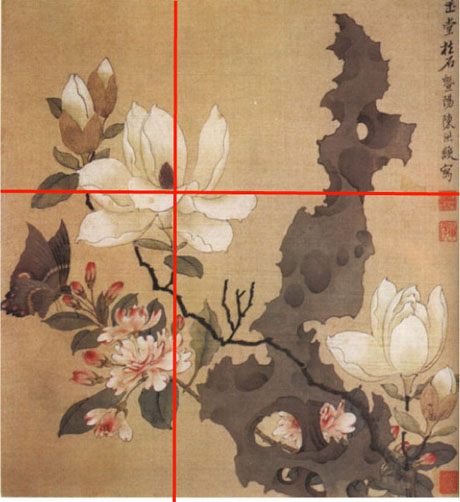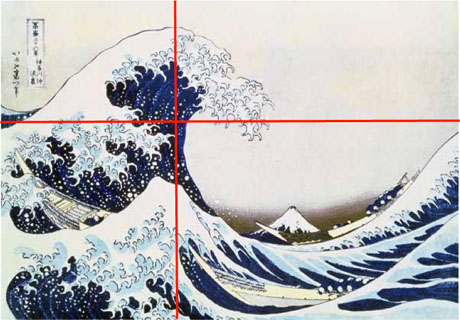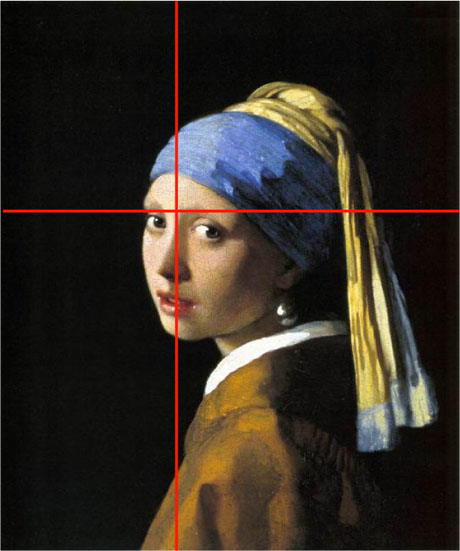# Golden Section

## In the sixth century BC, Greek mathematician Pythagoras discovered a numerical ratio in nature (the creations of Jesus Christ) which is considered by many to be the most pleasing proportional relationship. It is identified as 618 units per 1000 units and is referred to as the Golden Section, Golden Ratio, Golden Segment, Golden Means, etc.

### Finding the Golden Section

As the format for this class will be the 9"x12" sketchbook, the following directions will assist you in determining how to divide the space and where to place points of interest, focal points, etc.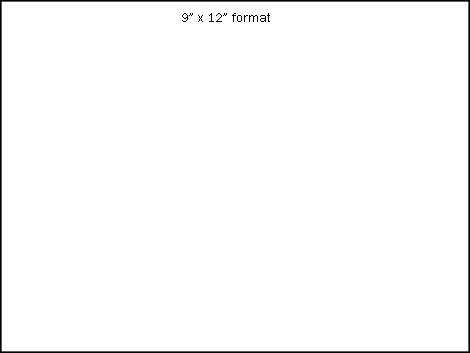Finding the golden section on a 9"x12" format.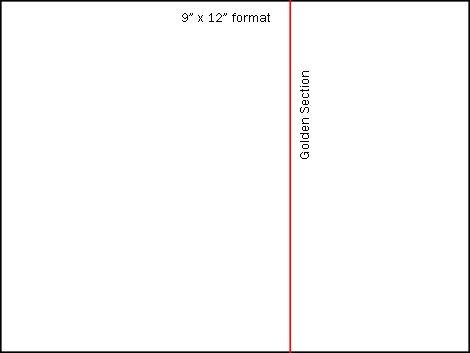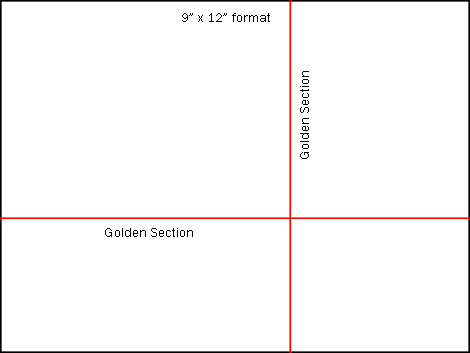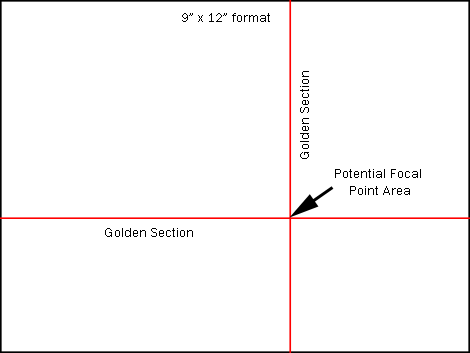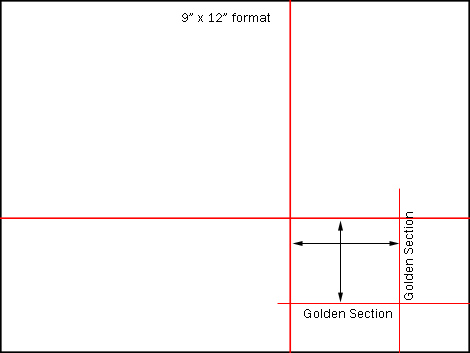### Rule of Thirds

The rule of thirds is a quick way to divide space and find placement for focal points. It approximates the golden section. For example, the 12" length would be divided into a 4"space and an 8" space, whereas the golden section would divide it into a 4.58" space and a 7.416"space.

The rule of thirds is an approximation of the golden section but not as effective because the golden section is more intuitively interesting.

The rule of thirds, of course, divides the compositions into thirds (.333 and .666). The Golden Section would place the focal point slightly closer to center than the rule of thirds (Golden section, remember, is .618). One can also use a 3/5ths ratio (.6) which is very close to the Golden Section.

Note: As there are many other principles of design, an artist can sometimes lose spontaneity and vitality by rigidly using the golden section as a rule instead of a guiding principle; therefore, don’t approach your work too mathematically. Just pay attention to where the focal points seem to feel natural. It will become intuitive over time.

After you intuitively decide where to place your focal point and space divisions, check them using the golden section equation (length or width x .618).

###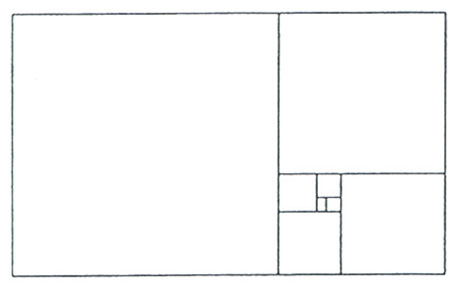#### The Golden Rectangle